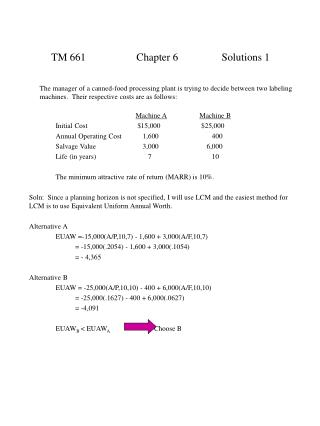DownloadDownload PresentationTM 661 Chapter 6 Solutions 1

TM 661 Chapter 6 Solutions 1

Download PresentationTM 661 Chapter 6 Solutions 1

- - - - - - - - - - - - - - - - - - - - - - - - - - - E N D - - - - - - - - - - - - - - - - - - - - - - - - - - -
Presentation Transcript

1. TM 661 Chapter 6 Solutions 1 The manager of a canned-food processing plant is trying to decide between two labeling machines. Their respective costs are as follows: Machine AMachine B Initial Cost \$15,000 \$25,000 Annual Operating Cost 1,600 400 Salvage Value 3,000 6,000 Life (in years) 7 10 The minimum attractive rate of return (MARR) is 10%. Soln: Since a planning horizon is not specified, I will use LCM and the easiest method for LCM is to use Equivalent Uniform Annual Worth. Alternative A EUAW =-15,000(A/P,10,7) - 1,600 + 3,000(A/F,10,7) = -15,000(.2054) - 1,600 + 3,000(.1054) = - 4,365 Alternative B EUAW = -25,000(A/P,10,10) - 400 + 6,000(A/F,10,10) = -25,000(.1627) - 400 + 6,000(.0627) = -4,091 EUAWB < EUAWA Choose B

2. TM 661 Chapter 6 Solutions 2 A \$250,000 piece of machinery is installed and is to be depreciated over 5 years. You may assume that the salvage value at the end of 5 years is \$ 0. The method of depreciation is to be 200% declining balance with conversion to straight line using the half-year convention (you may only deduct 1/2 year of depreciation in year 1). Establish a table showing the depreciation and the end of year book value for each year. Soln: DDB for year 1 = 250,000 x 0.4 = 100,000 x 1/2 = 50,000 DSl for year 1 = 250,000/5 = 50,000 x 1/2 = 25,000 DSL for year 2 = 200,000/4.5 = 44,444 t D200 DSL Bt 0 250,000 1 50,000 25,000 200,000 2 80,000 44,444 120,000 3 48,000 34,286 72,000 4 28,800 28,800 43,200 5 28,840 14,400 6 14,400 0

3. TM 661 Chapter 6 Solutions 3 A company is thinking of investing in a new filter for a smokestack. The project will cost \$750,000 which will be capitalized by investing \$500,000 internally and borrowing the remaining \$250,000. The loan is to be repaid over a 3 year period at 10% interest. Annual returns are expected to be \$200,000 per year with a \$100,000 salvage value at the end of year 5. The table below shows the project cash flow and loan repayment schedule. Complete the partial table below. MACRS deductions are based on a 3 year property class life. If the MARR is 20%, compute the Net Present Worth on an after tax cash flow basis.